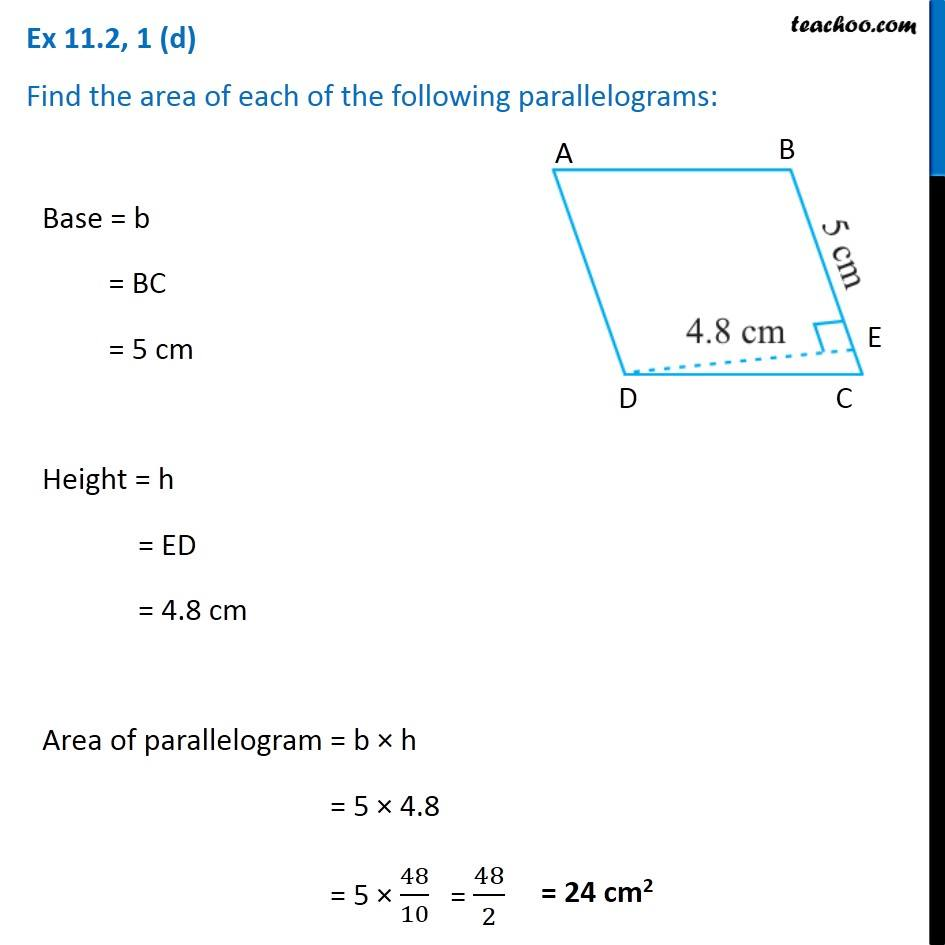Ex 11.2

Chapter 11 Class 7 Perimeter and Area
Serial order wiseIntroducing your new favourite teacher - Teachoo Black, at only ₹83 per month

### Transcript

Ex 11.2, 1 (d) Find the area of each of the following parallelograms: Base = b = BC = 5 cm Height = h = ED = 4.8 cm Area of parallelogram = b × h = 5 × 4.8 = 5 × 48/10 = 48/2